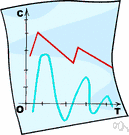topological space

(redirected from Vietoris topology)
Also found in: Thesaurus, Encyclopedia.

topological space

n.
A set of points together with a topology defined on them.

topological space

n
(Mathematics) maths a set S with an associated family of subsets τ that is closed under set union and finite intersection. S and the empty set are members of τ
ThesaurusAntonymsRelated WordsSynonymsLegend:
 Noun 1topological space - (mathematics) any set of points that satisfy a set of postulates of some kind; "assume that the topological space is finite dimensional"mathematical spaceinfinite, space - the unlimited expanse in which everything is located; "they tested his ability to locate objects in space"; "the boundless regions of the infinite"math, mathematics, maths - a science (or group of related sciences) dealing with the logic of quantity and shape and arrangementset - (mathematics) an abstract collection of numbers or symbols; "the set of prime numbers is infinite"subspace - a space that is contained within another spacenull space - a space that contains no points; and empty spacemanifold - a set of points such as those of a closed surface or an analogue in three or more dimensionsmetric space - a set of points such that for every pair of points there is a nonnegative real number called their distance that is symmetric and satisfies the triangle inequality
Translations
topologický prostor
topologisk rum